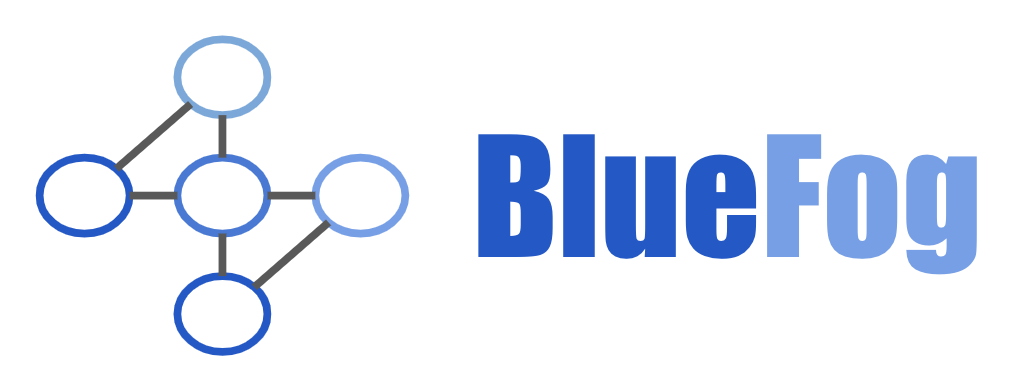# Bluefog¶Bluefog is a distributed training framework for PyTorch based on diffusion/consensus-type algorithm. The goal of Bluefog is to make distributed and decentralized machine learning fast, fault-tolerant, friendly to heterogeneuous environment, and easy to use.

The most distinguishable feature of Bluefog compared with other popular distributed training frameworks, such as DistributedDataParallel provided by pytorch, Horovod, BytePS, etc., is that our core implementation rooted on the idea that we introduce the virtual topology into the multiple processes and local averaging will converge to the global average as iteration keep going, where local averaging is defined based on the connection in the virtual topology.

Leveraging the One-sided Communication Ops (i.e. remote-memory access) of MPI, Bluefog is not only distributed but also decentralized training framework with high performance.

• Unlike the ring-allreduce based Bulk Synchronous Parallel algorithm, each process in bluefog is highly decoupled so that we can maximize the power of asynchronous algorithm.
• Unlike the parameter-server (PS) based architecture, there is no central node to collect and distribute information so that we can avoid the bottleneck problem existing in the PS.

The cost of those advantages is the inconsistence between models. Please check our papers to see the theoratical guarantee.

Important

Although the most torch-based APIs perform well, this repository is still in the early stage of development and more features are waiting to be implemented. If you are interested, you are more than welcome to join us and contribute this project!

## Quick Start¶

First, make sure your environment is with `python>=3.7` and openmpi >= 4.0. Then, install Bluefog with: `pip install --no-cache-dir bluefog`. Check the Installing Bluefog page if you need more information or other install options. We provide high-level wrapper for torch optimizer. Probably, the only thing you need to modify the existing script to distributed implementation is wrapping the optimizer with our `DistributedBluefogOptimizer`, then run it through `bfrun`. That is it!

```# Execute Python functions in parallel through
# bfrun -np 4 python file.py

import torch
import bluefog.torch as bf
...
bf.init()
optimizer = optim.SGD(model.parameters(), lr=lr * bf.size())
optimizer = bf.DistributedBluefogOptimizer(
optimizer, named_parameters=model.named_parameters()
)
...
```

We also provide lots of low-level functions, which you can use those as building blocks to construct your own distributed trainning algorithm. The following example illustrates how to run a simple consensus algorithm through bluefog.

```import torch
import bluefog.torch as bf

bf.init()
x = torch.Tensor([bf.rank()])
for _ in range(100):
x = bf.neighbor_allreduce(x)
print(f"{bf.rank()}: Average value of all ranks is {x}")
```

One noteable feature of Bluefog is that we leverage the One-sided Communication of MPI to build a real decentralized and asynchronized algorithms. This is another example about how to use Bluefog to implement an asynchronized push-sum consensus algorithm.

```import torch
import bluefog.torch as bf
from bluefog.common import topology_util

bf.init()

# Setup the topology for communication
bf.set_topology(topology_util.PowerTwoRingGraph(bf.size()))
outdegree = len(bf.out_neighbor_ranks())
indegree = len(bf.in_neighbor_ranks())

# Create the buffer for neighbors.
x = torch.Tensor([bf.rank(), 1.0])
bf.win_create(x, name="x_buff", zero_init=True)

for _ in range(100):
bf.win_accumulate(
x, name="x_buff",
dst_weights={rank: 1.0 / (outdegree + 1)
for rank in bf.out_neighbor_ranks()},
require_mutex=True)
x.div_(1+outdegree)
bf.win_sync_then_collect(name="x_buff")

bf.barrier()
# Do not forget to sync at last!
bf.win_sync_then_collect(name="x_buff")
print(f"{bf.rank()}: Average value of all ranks is {x/x[-1]}")
```

Please explore our examples folder to see more about how to implemented deep learning trainning and distributed optimization algorithm quickly and easily through bluefog. If you want to understand more on how to use the low-level API as the building blocks for your own distributed algorithm, please read our Bluefog Operations Explanation page.

INSTALLATION